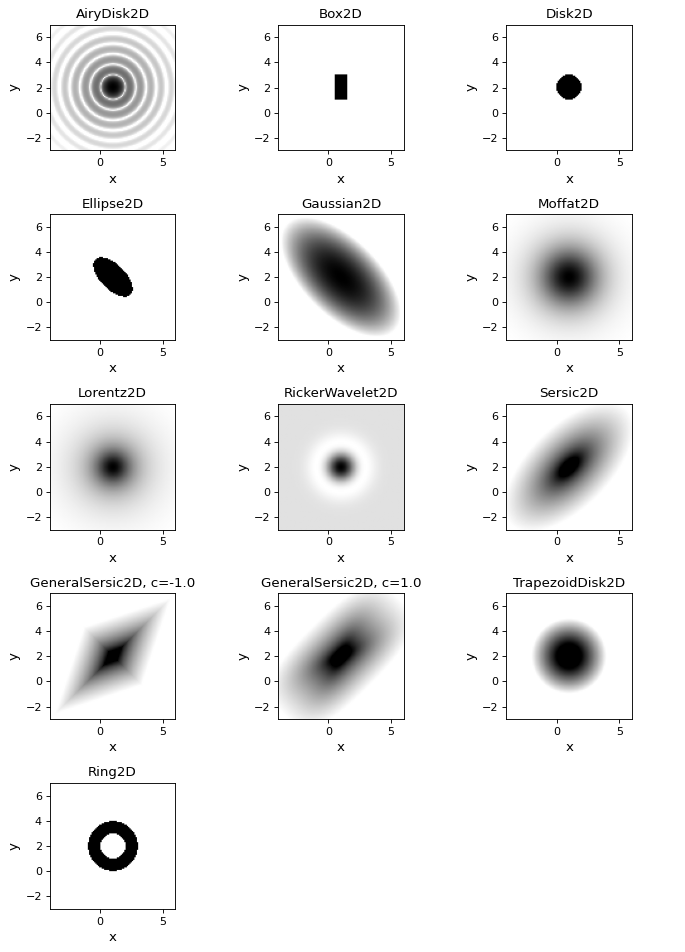2D Models¶

These models take as input x and y arrays.

Operations¶

These models perform simple mathematical operations.

• Const2D model returns the constant replicated by the number of input x and y values.

Shapes¶

These models provide shapes, often used to model general x, y, z data.

• Planar2D model computes a tilted plan with specified x,y slopes and z intercept

Profiles¶

These models provide profiles, often used sources in images. All models have parameters giving the x,y location of the center and an amplitude.

• AiryDisk2D model computes the Airy function for a radius

• Box2D model computes a box with x,y dimensions

• Disk2D model computes a disk a radius

• Ellipse2D model computes an ellipse with major and minor axis and rotation angle

• Gaussian2D model computes a Gaussian with x,y standard deviations and rotation angle

• Moffat2D model computes a Moffat with x,y dimensions and alpha (power index) and gamma (core width)

• RickerWavelet2D model computes a symmetric RickerWavelet function with the specified sigma

• Sersic2D model computes a Sersic profile with an effective half-light radius, rotation, and Sersic index

• TrapezoidDisk2D model computes a disk with a radius and slope

• Ring2D model computes a ring with inner and outer radii

(png, svg, pdf)